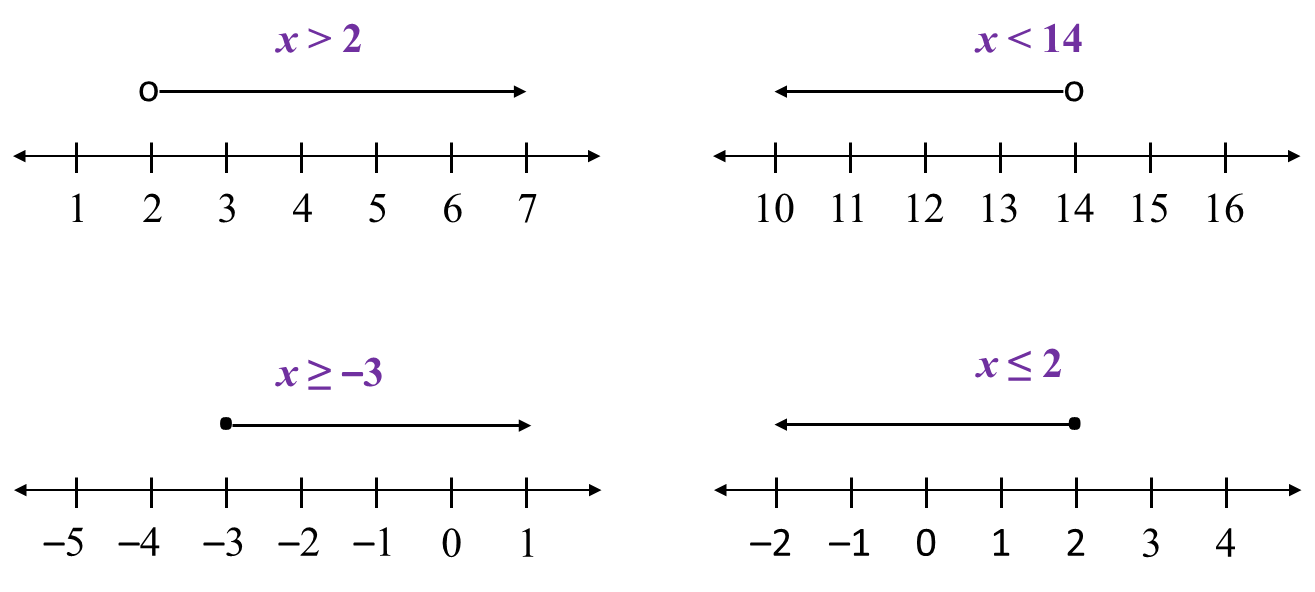# 7.1.1 Linear Inequalities

7.1 Linear Inequalities

7.1.1 Inequalities
1.   To write the relationship between two quantities which are not equal, we use the following inequality signs:
> greater than
< less than
≥ greater than or equal to
≤ less than or equal to

2.   7 > 4 also means 4 < 7.  7 > 4 and 4 < 7 are equivalent inequalities.

7.1.2  Linear Inequalities in One Unknown
1.   An inequality in one unknown to the power of 1 is called a linear inequality.
Example: 2x + 5 > 7

2.   A linear inequality can be represented on a number line.
Example:7.1.3 Computation on Inequalities
1.   When a number is added or subtracted from both sides of an inequality, the condition of the inequality is unchanged.
Example:
Given 5 > 3
Then, 5 + 2 > 3 + 2 ← (symbol ‘>’ remains)
Hence, 7 > 5

2.   When both sides of an inequality are multiplied or divided by the same positive number, the condition of the inequality is unchanged.
Example:
Given 4x ≤ 16
Then, 4x ÷ 4 ≤ 16 ÷ 4 ← (symbol ‘≤’ remains)
Hence, x ≤ 4

3.   When both sides of an inequality are multiplied or divided by the same negative number, the inequality is reversed.
Example:
Given –3 > –5
Hence, 3 < 5
Given –5y > –10
Then, –5y ÷ 5 > –10 ÷ 5
–y > –2
Hence, y < 2